occasional meanderings in physics' brave new world

## Friday, January 30, 2009

### Still Down South II

Now I'm back down south in Wanaka again, as another week comes to an end. A stable southerly offers good walking opportunities for the next few days, and there are dogs to walk. Thank you to all those people who have given me support recently. Hopefully a visa will arrive soon. If not ... AF will not be silent.

## Tuesday, January 27, 2009

### Quote of the Week

Thanks to David Corfield for this survey paper (on foundational categorical topology) from the mathematician Paul Taylor. Breaking my personal rule of mostly ignoring criticism of physicists from mathematicians, I put here the following gem of a quote.
Physics very probably relies on compactness of the interval, but I would be very sceptical if you told me that some property of black holes depends on excluded middle. Have you actually developed the analogous constructive theory, and found observational evidence to distinguish it from the classical one? This is, after all, what the experimental method says that you should do.

## Sunday, January 25, 2009

### M Theory Lesson 256

Larger products of MUB operators also lead to nice magic matrices. For example, consider this stunning exact magic matrix: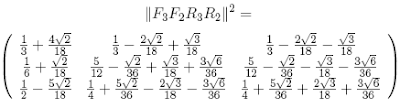which has numerical values roughly equal to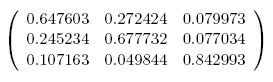Observe that this matrix is similar in form to, although not the same as, the CKM quark mixing matrix.

## Friday, January 23, 2009

### M Theory Lesson 255

The numbers appearing in the absolute value of $R_3 R_2$ also occur in other MUB combinations of both three dimensional and two dimensional operators. For instance, with an $R_2$ factor on the right, we obtainwhereas an $F_2$ factor recovers yet another form of the tribimaximal mixing matrix:## Thursday, January 22, 2009

### Still Down South

As the nice lady at Air New Zealand said to me, in sympathy at my status as an undesirable alien, at least I can enjoy more of the summer. My little cabin in the garden has been very comfortable. But next week it is probably time to move on, so if no visa appears I will head down south again to Wanaka, from where it is easy enough to catch a bus back to Christchurch.## Wednesday, January 21, 2009

### M Theory Lesson 254

Dave at Carl's blog has pointed out that

$\textrm{cos} (\theta) = \frac{2 \sqrt{2}}{\sqrt{9}}$

where $\theta$ is the sum of row (or column) entries in the circulant decomposition of the tribimaximal mixing matrix. In M Theory, we are used to coming across this number $2/9$. For example, it appears as a determinant,

$\frac{2}{9} = 1 - (\frac{1}{\sqrt{3}} + \frac{2}{3} i)(\frac{1}{\sqrt{3}} - \frac{2}{3} i)$

in the 2D component of the transform of the tribimaximal mixing matrix. The number

$\frac{1}{\sqrt{3}} + \frac{2}{3}$

also appears in the 2D component of $| R_3 R_2 |$, a MUB analogue of the (absolute value of) the tribimaximal matrix. That is, this number is a normalised form of $(1 + i \omega^2)$, where $\omega$ is the usual cubed root of unity.

## Monday, January 19, 2009

### CKM Rules V

Recall that the neutrino tribimaximal mixing matrix is most easily expressed in the form $F_3 F_2$, the product of two quantum Fourier operators. One wonders whether or not the other Combescure MUB operators can be combined to give us some information about the CKM matrix. For example, we have thatObserve that the absolute value of this matrix takes the form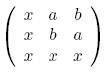where all entries are real, and the square of this absolute value looks like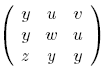The cube finally takes a form reminiscent of the CKM, with all entries possibly distinct. In this example, the values of $a$, $b$ and $x$ are given by $\sqrt{2 + \sqrt{3}}$, $\sqrt{2 - \sqrt{3}}$ and $\sqrt{2}$.

## Sunday, January 18, 2009

### Keaaawww

This photo is only slightly modified:### M Theory Lesson 253

One zeta type function for a matrix $A$ is given by

$\zeta_A (s) = \textrm{det} (I - \frac{A}{s})$.

For the Weyl circulant $A = (234 \cdots n1)$ in odd prime dimension this takes the form

$\zeta_A (s) = 1 - s^{-p}$

and so the product of all such determinants would look like

$\prod_p (1 - s^{-p})$,

which is strangely similar to the (inverse of the) usual zeta Euler product, except that $p$ and $s$ are interchanged. Observe that this product takes the form

$\prod_p (1 - s^{-p}) = \sum_n \mu (n) s^{- \kappa (n)}$

where $\mu (n)$ is the Mobius function and $\kappa (n)$ is the sum of the prime factors of $n$, otherwise known as the sequence A001414, or the integer logarithm of $n$.

## Saturday, January 17, 2009

### M Theory Lesson 252

In vector space arithmetic, instead of ordinary products and additions we have something like tensor products and direct sums. For example, the combination of circulant primes in a matrix ordinal $n$ is a product.

In a paper on random permutation matrices Hambly et al remind us of the observation of Wieand, that the spectrum of such a matrix, although different, has some surprising similarities to that of a random unitary matrix. But the basic Weyl circulant is only one of the MUB factors, coming from the Fourier transform. Combinations of braided (MUB) 1-circulants, with entries that are roots of unity, give rise to more general large complex matrices with one entry in each row and column. Since the eigenvalues of MUB circulants are also always on the unit circle (they are roots of unity) it would be interesting to study the behaviour of the eigenvalues of their products.

Aside: Carl's new Koide paper has many interesting things to say about circulants.

## Friday, January 16, 2009

### M Theory Lesson 251

Bob Coecke reminds us that his 2008 paper on bases and quantum protocols also uses oriented trivalent vertices. That's nice, because we knew that the Pauli MUB was associated to the trefoil quandle, and now it is associated at a more fundamental level with the Khovanov cube.

The question is, how does this correspondence extend to higher dimensional MUBs? For odd prime dimensions, the MUBs may be specified by the eigenvector operators, namely (a) the Fourier operator and (b) the sequence of Combescure circulants $M_1, M_2, \cdots, M_n$, which includes the identity. The corresponding MUB matrices (which are diagonalised by the eigenvector operators) are (a) the Weyl 1-circulant $(234 \cdots n1)$ (generating the permutation group $S_n$) and (b) a sequence of braided circulants that use the diagonal Weyl operator $D$. Each of the $n(n+1)$ pairs of MUB matrices forms a quantum plane with rules $A_i A_j = \omega^k A_j A_i$, for $\omega$ the $n$-th root of unity.

In particular, the Weyl 1-circulant $C$ acts on the other $n$ matrices $A_i$ to cycle them, just as the Pauli $\sigma_X$ acts on either $\sigma_Z$ or $\sigma_Y$ to yield the other operator. This special behaviour of the Fourier MUB component marks one edge of a (potential) Tutte graph, which we may consider a root in the case of trees. If, in fact, trees are matched to the MUB sequence, this results in an operadic labelling of the MUB index $n$.

## Wednesday, January 14, 2009

### M Theory Lesson 250

Recall that the Tutte polynomial for the trefoil knot is based on the trivalent vertex graph. On the other hand, we would like to consider categorified invariants such as in Khovanov homology.

The Khovanov cube for the trefoil knot contains the 8 ($= 2^3$) possible smoothing diagrams for the trefoil. For each crossing piece of the original knot there are two ways to smooth the crossing. In other words, there are two types of edge on the Tutte graphs. We might as well label these two colours by inward and outward arrows. Then the eight vertices of a cube are given by the diagrams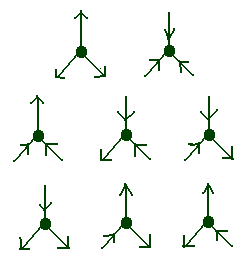## Monday, January 12, 2009

### M Theory Lesson 249

To be definite, rather than turtles, let us pick the Weyl 1-circulant $(234 \cdots n1)$ all the way down through the prime factors of a number $n$. Then for the number $6$, the two possible permutations $P \in S_6$ areboth of which satisfy $P^6 = 1$. For more than two prime factors for $n$, this process still selects a permutation satisfying $P^n = 1$, such as in this examplefor $12 = 3 \times 2 \times 2$. The product of an infinite number of prime factors would result in an infinite matrix.

## Sunday, January 11, 2009

### Riemann Products III

It is known that the value of $\zeta (s)$ at $s = 0$ is $\zeta (0) = - 1/2$. On the other hand, recall that the Everything Seminar showed us that

$1 - 1 + 1 - 1 + 1 - 1 + 1 - \cdots = \frac{1}{1 + 1} = \frac{1}{2}$ and
$1 + 1 + 1 + 1 + 1 + 1 + 1 + \cdots = - \frac{1}{2}$

so that the product for $1/ \zeta(s)$ tells us that

$1/ \zeta(0) = 1 - 1[1 + 1 + 1 + \cdots]$
$+ 1[1(1 + 1 + 1 + \cdots) + 1(1 + 1 + 1 + \cdots) + 1(1 + 1 + 1 + \cdots) + \cdots] - \cdots$
$= 1 + \frac{1}{2} + \frac{1}{4} + \cdots = 2$,

which is almost the right answer! What does a minus sign matter, anyway? We can probably fix that. Similarly, the Pauli product expression indicates that

$\frac{1}{\zeta (-1)} = 1 - 2 - 3 - 5 + 6 - 7 + 10 + \cdots = -12$

somehow! At least thinking of complex numbers as infinite sums is much nicer than messing around with standard analysis.

### Riemann Products II

The consideration of ordinals $N = p_1 p_2 p_3 \cdots p_k$, where all prime factors $p_i$ are distinct, occurs as the Pauli exclusion principle for the Riemann gas, whose partition function is the Riemann zeta function.

The product expression for the inverse zeta function is always well defined for finite products, which define a sequence of functions $\zeta_N$ for $N$ such a Pauli ordinal. Showing that the limit $N \rightarrow \infty$ leads to a well defined zeta function for basically all $s$ values is equivalent to the Riemann hypothesis.

## Saturday, January 10, 2009

### Riemann Products

Speaking of Euler products associated to the zeta function, the totient function leads one to consider the product

$\prod_p (1 - \frac{1}{p})(1 - \frac{1}{p^s}) = \prod_p [(1 - \frac{1}{p}) - \frac{1}{p^s}(1 - \frac{1}{p})]$
$= \prod_p (1 - \frac{1}{p})[1 - \sum_{p_1} \frac{1}{p_{1}^{s}} + \sum_{p_1,p_2} \frac{1}{(p_1 p_2)^s} - \cdots ]$

where one of the terms on the right hand side is a sum over $k$ distinct prime factors. That is, in cancelling the $\phi(\infty)$ factors, we have that

$\frac{1}{\zeta (s)} = 1 + \sum_k (-1)^k \sum_{p_1,p_2, \cdots, p_k} \frac{1}{(p_1p_2 \cdots p_k)^s}$

which is a simple sum over all ordinals $n$ composed of single prime factors. This may be rewritten

$\frac{1}{\zeta (s)} = \sum_{even} \frac{1}{n^s} - \sum_{odd} \frac{1}{n^s} = \sum_n \frac{\mu (n)}{n^s}$

where the parity counts the number of prime factors in $n$, and $1$ is an even prime. The function $\mu (n)$ is the Mobius function, which is zero for $n$ with repeated prime factors.

### M Theory Lesson 248

In lesson 226 we wondered about prime dimensions for which the allowed MUB matrix entries formed a finite field on a prime number of elements. These are precisely the primes of Sophie Germain, that is $p$ such that $2p + 1$ is also prime. Sophie Germain studied them in connection with Fermat's Last Theorem, in particular for the case $x^5 + y^5 = z^5$.

Now let $\phi (n)$ be the number of integers less than or equal to $n$ which are coprime to $n$. This is the totient function of Euler. Germain primes are the primes that solve an equation of the form $\phi (n) = 2p$. The totient function tells us the cardinality of the multiplicative group of integers modulo $n$. It may be expressed as

$\phi (n) = n \prod_{p | n} (1 - \frac{1}{p})$

and thus it is related to the Riemann zeta function by

$\sum_{n=1}^{\infty} \frac{\phi (n)}{n^s} = \frac{\zeta (s-1)}{\zeta (s)}$.

Note that the Germain primes define a subsequence of the $\phi (n)$, and of the prime numbers, which one might view as a natural regularization of the zeta function.

## Friday, January 09, 2009

### Oh, Pythagoras

In Simon Singh's wonderful book on Fermat's Last Theorem, he tells of the death of Pythagoras of Samos:
Following the death of their founder and the attack by Cylon, the Brotherhood left Croton for other cities in Magna Graecia, but the persecution continued and eventually many of them had to settle in foreign lands. This enforced migration encouraged the Pythagoreans to spread their mathematical gospel throughout the ancient world...
It is difficult to murder ideas. Most attempts to do so fail.

## Thursday, January 08, 2009

### M Theory Lesson 247

A simple way to express a cardinal number $n$ as a dimension is to write $n$ as the $n$ dimensional identity matrix. In this case, the natural way to express prime factorizations is in terms of embedded identity matrices. For example, since $6 = 2 \times 3$ there are two ways to write down a circulant permutation such that in the first case $C^3 = 1$ and in the second case $C^2 = 1$.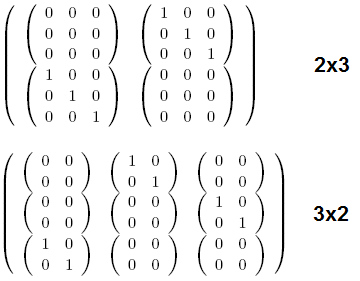In other words, the factorization of the number 6 results in roots of unity of order less than 6. In general, one embeds identities into the basic Fourier circulant $(234...n1)$. Since matrix elements are noncommutative, one would not dream of using classical geometry to study categorified arithmetic.